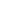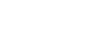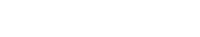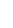# Fineness Modulus Method of Concrete Mix DesignReading time: 1 minute

## Fineness Modulus Method of Concrete Mix Design

The index number, which describes the relative sizes of coarse and fine aggregates, is called as fineness modulus (FM). Fineness modulus is determined separately by sieving coarse and fine aggregates through the following set of sieve. IS sieves for coarse aggregates are 80 mm, 40 mm, 20 mm, 10 mm and 4.75 mm. IS sieves for fine aggregates are 1 mm, 600, 300, 212, 150, and 75.Where, X= Fineness modulus of coarse aggregate, Y= Fineness modulus of fine aggregates, and Z= Economical value of fineness modulus for combined aggregate. The above formula will fix the proportion of fine aggregate to coarse aggregate. The percentage of the coarse aggregate is equal to 100 minus the percentage of fine aggregate. Table below gives the economical value of F.M. for combined aggregate.

### Table: Economical Value of F.M. for Combined Aggregate

 S. No. Particle size (mm) Fineness modulus Average F.M. Minimum Maximum 1 20 4.7 5.1 4.90 2 25.4 5.0 5.5 5.25 3 31.5 5.2 5.7 5.45 4 38.1 5.4 5.9 5.65 5 40 5.7 6.3 6.00 6 80 6.5 7.0 6.75
With high water-cement ratio, lower value of fineness modulus should be adopted and with low water-cement ratio, higher value of fineness modulus should be adopted.The next step is to find out the quantity of water-cement paste to be added to fine and coarse aggregates in order to obtain the required workability. Slump test is used to confirm the quantity of water-cement paste. The method consists of preparing a number of batches of combined aggregate in the fixed proportions. In each batch, varying quantities of water-cement paste having fixed water-cement ratio are added to get the required slump. In order to start with, the minimum quantity of water is added in the first batch. It is determined from the following formula:where, P= Quantity of cement by weight, Y= Quantity of fine aggregate by weight, Z= Quantity of coarse aggregate by weight, and= Water cement ratio